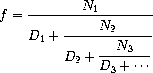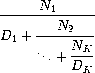Diagnostics are meant for you to check your understanding of the week's material. They are not worth any points and are not counted towards midterm recovery points; instead, diagnostics are solely for your benefit. If you find yourself struggling with the questions in this diagnostic, consider signing up for Tutoring.

## Recursion

### Question 1: In summation...

Write the recursive version of `summation`, which takes two arguments, a number `n` and a function `term`, applies `term` to every number between `1` and `n` inclusive, and returns the sum of those results.

``````def summation(n, term):
"""Return the sum of the 0th to nth terms in the sequence defined
by term.
Should be implemented using recursion.

>>> summation(5, lambda x: x * x * x)
225
>>> summation(9, lambda x: x + 1)
55
>>> summation(5, lambda x: 2**x)
63
"""
if n == 0:
return term(0)
return term(n) + summation(n - 1, term)``````

### Question 2

Write a function `has_seven` that takes a positive integer `n` and returns whether `n` contains the digit 7. Do not use any assignment statements - use recursion instead:

``````def has_seven(k):
"""Returns True if at least one of the digits of k is a 7, False otherwise.

>>> has_seven(3)
False
>>> has_seven(7)
True
>>> has_seven(2734)
True
>>> has_seven(2634)
False
>>> has_seven(734)
True
>>> has_seven(7777)
True
"""
if k % 10 == 7:
return True
elif k < 10:
return False
else:
return has_seven(k // 10)``````

### Question 3

An infinite continued fraction is an expression of the form:As an example, one can show that the inverse of the golden ratio can be computed by setting all of the terms to 1. A way to approximate the value of an infinite continued fraction is to compute the value of truncating after a given number of terms. This truncation, called the `k`-term finite continued fraction, has the form:Write a function `iterative_continued_frac`, which takes two functions `n_term` and `d_term`, which each produce the `ith` `N` and `D` term respectively, and a number `k` and returns the `k`-term finite continued fraction. Use iteration to perform the computation.

``````def iterative_continued_frac(n_term, d_term, k):
"""Returns the k-term continued fraction with numerators defined by n_term
and denominators defined by d_term.

>>> # golden ratio
... round(iterative_continued_frac(lambda x: 1, lambda x: 1, 8), 3)
0.618
>>> # 1 / (1 + (2 / (2 + (3 / (3 + (4 / 4))))))
... round(iterative_continued_frac(lambda x: x, lambda x: x, 4), 6)
0.578947
"""
result, i = 0, k
while i >= 1:
result, i = n_term(i) / (d_term(i) + result), i-1
return result``````

Now write a function `recursive_continued_frac` that uses recursion to compute the `k`-term finite continued fraction. Hint: try writing a recursive helper function to do most of the work, rather than trying to do the recursion with `recursive_continued_frac` directly.

``````def recursive_continued_frac(n_term, d_term, k):
"""Returns the k-term continued fraction with numerators defined by n_term
and denominators defined by d_term.

>>> # golden ratio
... round(recursive_continued_frac(lambda x: 1, lambda x: 1, 8), 3)
0.618
>>> # 1 / (1 + (2 / (2 + (3 / (3 + (4 / 4))))))
... round(recursive_continued_frac(lambda x: x, lambda x: x, 4), 6)
0.578947
"""
return recursive_continued_frac_helper(n_term, d_term, k, 1)

def recursive_continued_frac_helper(n_term, d_term, k, i):
denom = d_term(i)
if i < k:
denom += recursive_continued_frac_helper(n_term, d_term, k, i+1)
return n_term(i) / denom``````

## Tree recursion

### Question 4: Partition

The number of partitions of a positive integer `n` is the number of ways in which `n` can be expressed as the sum of positive integers in increasing order. For example, the number `5` has `7` partitions.

``````5 = 5
5 = 1 + 4
5 = 2 + 3
5 = 1 + 1 + 3
5 = 1 + 2 + 2
5 = 1 + 1 + 1 + 2
5 = 1 + 1 + 1 + 1 + 1``````

Write a tree-recursive function `part(n)` that returns the number of partitions of `n`.

Hint: Introduce a helper function that computes partitions of `n` using only a subset of the integers less than or equal to `n`. Once you have done so, you can use very similar logic to the `count_change` function from the lecture notes:

``````def part(n):
"""Return the number of partitions of positive integer n.

>>> part(5)
7
>>> part(10)
42
>>> part(15)
176
>>> part(20)
627
"""
return part_max(n, n)

def part_max(n, m):
"""Return the number of partitions of n using integers up to m.

>>> part_max(5, 3)
5
"""
if n < 0 or m <= 0:
return 0
if n == 0:
return 1
return part_max(n-m, m) + part_max(n, m-1)``````

### Question 5

Write a function that takes a positive integer `n` and returns the number of ten-pairs it contains. A ten-pair is a pairs of digits within `n` that sum to 10. Do not use any assignment statements.

The number 7,823,952 has 3 ten-pairs. The first and fourth digits sum to 7+3=10, the second and third digits sum to 8+2=10, and the second and last digit sum to 8+2=10:

``````def ten_pairs(n):
"""Return the number of ten-pairs within positive integer n.

>>> ten_pairs(7823952)
3
>>> ten_pairs(55055)
6
>>> ten_pairs(9641469)
6
"""
if n < 10:
return 0
else:
return ten_pairs(n//10) + count_digit(n//10, 10 - n%10)

def count_digit(n, digit):
"""Return how many times digit appears in n.

>>> count_digit(55055, 5)
4
"""
if n == 0:
return 0
else:
if n%10 == digit:
return count_digit(n//10, digit) + 1
else:
return count_digit(n//10, digit)``````

## Orders of Growth

### Question 6

``````def bar(n):
i, sum = 1, 0
while i <= n:
sum += biz(n)
i += 1
return sum

def biz(n):
i, sum = 1, 0
while i <= n:
sum += i**3
i += 1
return sum``````

O(n2)

### Question 7

``````def bonk(n):
sum = 0
while n >= 2:
sum += n
n = n / 2
return sum``````

O(log(n))